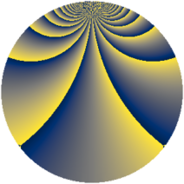# Properties

 Label 2001.2.bcLevel $2001$ Weight $2$ Character orbit 2001.bc Rep. character $\chi_{2001}(47,\cdot)$ Character field $\Q(\zeta_{28})$ Dimension $2640$ Sturm bound $480$

# Related objects

## Defining parameters

 Level: $$N$$ $$=$$ $$2001 = 3 \cdot 23 \cdot 29$$ Weight: $$k$$ $$=$$ $$2$$ Character orbit: $$[\chi]$$ $$=$$ 2001.bc (of order $$28$$ and degree $$12$$) Character conductor: $$\operatorname{cond}(\chi)$$ $$=$$ $$87$$ Character field: $$\Q(\zeta_{28})$$ Sturm bound: $$480$$

## Dimensions

The following table gives the dimensions of various subspaces of $$M_{2}(2001, [\chi])$$.

Total New Old
Modular forms 2928 2640 288
Cusp forms 2832 2640 192
Eisenstein series 96 0 96

## Trace form

 $$2640q + O(q^{10})$$ $$2640q - 24q^{10} - 6q^{12} + 20q^{15} + 440q^{16} + 34q^{18} - 36q^{21} - 24q^{24} - 440q^{25} - 24q^{27} - 200q^{30} + 12q^{36} - 56q^{37} - 4q^{39} + 72q^{40} + 8q^{43} - 64q^{45} + 22q^{48} - 392q^{49} - 24q^{52} + 24q^{54} - 328q^{55} - 308q^{58} - 48q^{60} + 72q^{61} - 252q^{64} + 28q^{66} + 104q^{70} - 72q^{73} + 240q^{75} - 88q^{76} + 184q^{78} + 120q^{79} + 64q^{81} + 208q^{82} - 36q^{84} + 48q^{85} + 96q^{87} + 464q^{88} - 104q^{90} + 112q^{93} - 112q^{94} + 350q^{96} - 120q^{97} - 196q^{99} + O(q^{100})$$

## Decomposition of $$S_{2}^{\mathrm{new}}(2001, [\chi])$$ into newform subspaces

The newforms in this space have not yet been added to the LMFDB.

## Decomposition of $$S_{2}^{\mathrm{old}}(2001, [\chi])$$ into lower level spaces

$$S_{2}^{\mathrm{old}}(2001, [\chi]) \cong$$ $$S_{2}^{\mathrm{new}}(87, [\chi])$$$$^{\oplus 2}$$